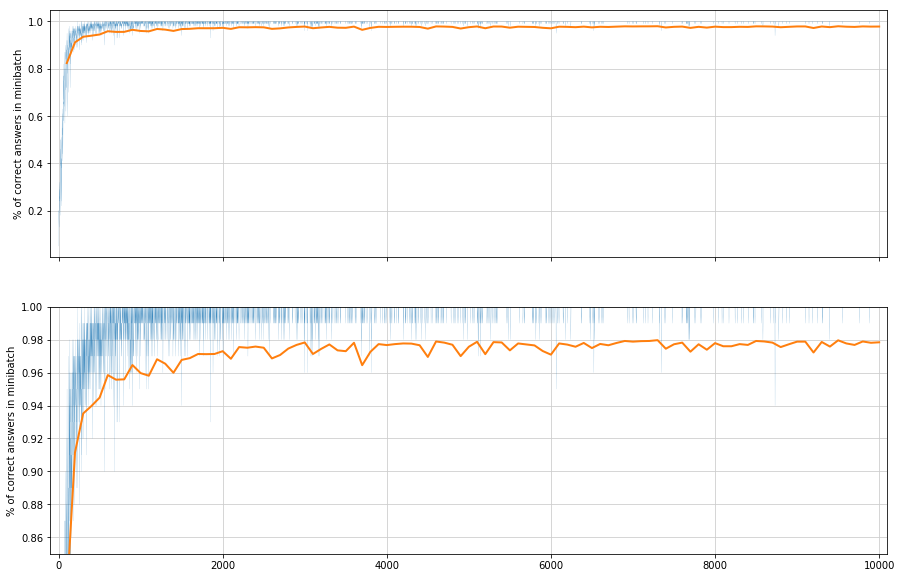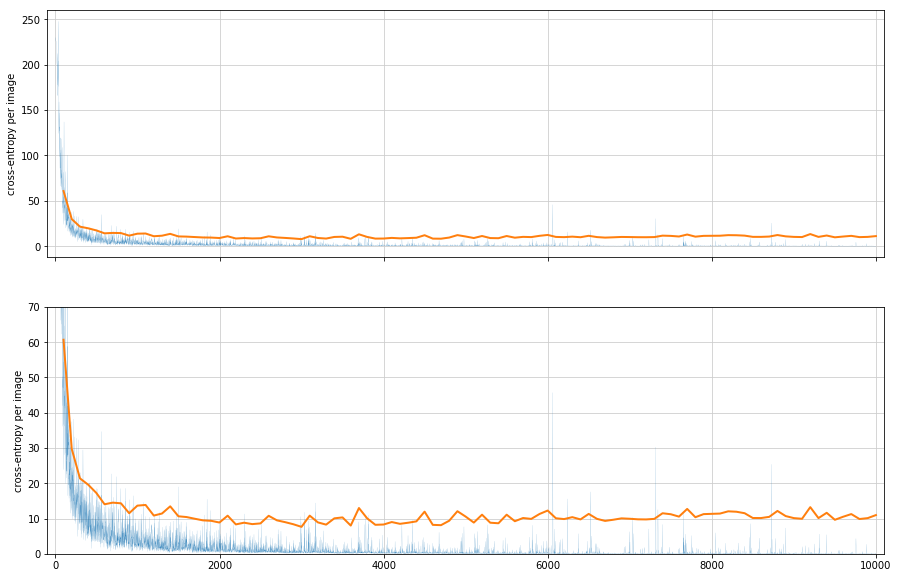## Introduction¶

This notebook is a continuation in a series (part 1, part 2) that follows Martin Gorner's session on deep learning (youtube, slide deck, google blog). It's very accessible, even for beginners, and I encourage you to watch it.

In part 2, we created a 5-layer network hoping that more degrees of freedom would improve our accuracy. While it did improve slightly, we can actually do better by simply changing the activation function!

In this notebook, I replace the sigmoid activation function with a rectified linear unit, more commonly known as ReLU, to help improve our 5-layer neural network. Most parts of this notebook will be similar to the 5-layer example, but I will point out where the key differences arise.

In :
```# Show matplotlib output within the notebook
%matplotlib inline
```
In :
```# Required packages are tensorflow, numpy, and matplotlib
import tensorflow as tf
from tensorflow.examples.tutorials.mnist import input_data
import matplotlib.pyplot as plt
import numpy as np
```
In :
```# Download the mnist dataset and save it to MNIST_data
# Initialize an mnist object with image labels converted into one-hot encoding (5 is [0, 0, 0, 0, 0, 1, 0, 0, 0, 0])
mnist = input_data.read_data_sets("MNIST_data/", one_hot=True)
```
```Extracting MNIST_data/train-images-idx3-ubyte.gz
Extracting MNIST_data/train-labels-idx1-ubyte.gz
Extracting MNIST_data/t10k-images-idx3-ubyte.gz
Extracting MNIST_data/t10k-labels-idx1-ubyte.gz
```
In :
```# Test if mnist loaded
plt.imshow(mnist.train.next_batch(1).reshape(28,28), cmap='gray')
```
Out:
`<matplotlib.image.AxesImage at 0x181caaa940>`## Initialize the variables and the biases¶

In :
```# Create a tensor for the samples with batch size (None because it is unknown at this time),
# Dimensions of the grayscale image: (28, 28)
# Number of channels: 1 because grayscale)

# In the video the expected input array was a (28, 28) because images are 28x28 pixels
# But by default, input_data.read_data_sets() already flattens this into a single row of 784 (because 28*28 = 784)

# X = tf.placeholder(tf.float32, [None, 28, 28, 1])  # 28,28 input
X = tf.placeholder(tf.float32, [None, 784])          # 784,1 input
```

### Set a small standard deviation to get random floats with values close to zero¶

In :
```# Create 5 tensors for weights of each of the 5 layers
# Each layer will have an associated bias that will be broadcasted to all weights
# This means that the bias tensor should be equal to the number of neurons in the layer

# First layer, 200 neurons
K = 200
W1 = tf.Variable(tf.truncated_normal([784, K], stddev=0.1))
B1 = tf.Variable(tf.zeros([K]))

# Second layer, 100 neurons
L = 100
W2 = tf.Variable(tf.truncated_normal([K, L], stddev=0.1))
B2 = tf.Variable(tf.zeros([L]))

# Third layer, 60 neurons
M = 60
W3 = tf.Variable(tf.truncated_normal([L, M], stddev=0.1))
B3 = tf.Variable(tf.zeros([M]))

# Fourth layer, 30 neurons
N = 30
W4 = tf.Variable(tf.truncated_normal([M, N], stddev=0.1))
B4 = tf.Variable(tf.zeros([N]))

# Output layer, 10 outputs
W5 = tf.Variable(tf.truncated_normal([N, 10], stddev=0.1))
B5 = tf.Variable(tf.zeros())
```

## Create the model¶

### Here we replace `tf.nn.sigmoid` with `tf.nn.relu`¶

In :
```# Model
# Yx = relu(X.W + b)
# Y = softmax(X.W + b)
#
# Variable  Explanation, tensor shape in []
# --------  -------------------------------
# Y       : predictions, Y[100,10]
# relu    : activation function and will be applied line-by-line, values will range from [0,inf)
# softmax : activation function and will be applied line-by-line, ensures all values in a vector will sum to 1.0
# X       : image tensor, X[100, 784], minibatches of 100
# W       : weights, W[784,10], "." between X and W means matrix multiply
# b       : biases, b

# Instead of having just one layer, we now have 5
# To connect the layers, use the output of the preceding layer as the input to the next
# The first input will be the image vector, and the final output will be the prediction in one-hot encoding
# For our layers, we use a relu activation function

# What is interesting with relu is that for input values less than zero, the output value will always be zero
# while for inputs greater than zero, it follows a linear pattern
# This helps resolve gradients better compared to the sigmoid function
Y1 = tf.nn.relu(tf.matmul(X, W1) + B1)
Y2 = tf.nn.relu(tf.matmul(Y1, W2) + B2)
Y3 = tf.nn.relu(tf.matmul(Y2, W3) + B3)
Y4 = tf.nn.relu(tf.matmul(Y3, W4) + B4)

# We use the softmax function for our output to make sure all the values will sum to 1.0
# You can consider each value as a probability the model assigns to the index that it is the right answer
# For example, [0, 0.2, 0, 0, 0, 0, 0, 0.9, 0, 0] means the model thinks
# the image it saw is 1 with probability 0.2 and 7 with probability 0.9
Y = tf.nn.softmax(tf.matmul(Y4, W5) + B5)

# Placeholder for correct answers in one-hot encoding
# These are known values to train with. Here, we use the label of each image
Y_ = tf.placeholder(tf.float32, [None, 10])
```

## Train using gradient descent¶

### A constant is added to in `tf.log` in computing the cross entropy to avoid `log(0)`¶

In :
```# Loss function
# We use cross-entropy to as a measure to compare our prediction with the known value
cross_entropy = -tf.reduce_sum(Y_ * tf.log(Y + 1e-10))  # we add a small constant to make sure our we never get log(0)

# Below is from the tutorial https://www.tensorflow.org/versions/r1.1/get_started/mnist/beginners
# tf.reduce_mean makes the cross-entropy value robust to changes in batch size.
# This means that you can keep the learning rate the same even if the batch size changes.
# cross_entropy = tf.reduce_mean(-tf.reduce_sum(Y_ * tf.log(Y + 1e-10), reduction_indices=))

# To train the neural network, we want to minimize cross-entropy between our predictions and the known values
# We use stochastic gradient descent to help us find the minimum

# To make sure we actually get close to the minimum, and not constantly overshoot it,
# we scale the gradient by a factor called the learning rate.
# Try experimenting by using different learning rates like 0.1, 0.03, 0.0005
optimizer = tf.train.GradientDescentOptimizer(0.003)

# The objective of the optimizer is to minimize the cross entropy
train_step = optimizer.minimize(cross_entropy)
```

## Success metrics¶

In :
```# This part is optional and has nothing to do anymore with training a neural network
# This is solely for reporting statistics to track progress

# Compares he position with the highest values are equal in the predictions and the labels
# Remember that we are using one-hot encoding for both, so we use tf.argmax to find the positions in the vectors
is_correct = tf.equal(tf.argmax(Y, 1), tf.argmax(Y_,1))

# % of correct answers found in the batch
accuracy = tf.reduce_mean(tf.cast(is_correct, tf.float32))
```

## Start training with TensorFlow¶

In :
```# Initialize all the variables and placeholders declared previously
# Remember that tensorflow does not immediately execute commands, but instead builds a representation first
# This part create a representation of the initialization process

# init = tf.initialize_all_variables()  # This method is now deprecated
init = tf.global_variables_initializer()
```
In :
```# To actually execute commands, we have to create a tensorflow session
sess = tf.Session()

# Pass init to actually initialize
sess.run(init)
```
In :
```# This part is not in the video.
# I use these lists to collect statistics to report later, similar to Martin's real-time charts in the video

# Statistics using training data
train_accuracy = []
train_cross_entropy = []

# Using testing data, which the neural network has never seen before
test_accuracy = []
test_cross_entropy = []
```
In :
```# There are 60,000 images in the MNIST training set
# Looping over 10000 times and retrieving 100 images at every iteration means that
# we would be able to use the entire training set at least once.
# Going over the entire training set means we have achieved 1 epoch
iterations = 10000
batch_size = 100

for i in range(1, iterations+1):
# Load batch of images and correct answers (labels)
batch_X, batch_Y = mnist.train.next_batch(batch_size)

# Train using train_step
# Remember to pass data to the placeholders X and Y_ by using a dictionary
# X is the training data in [100,784,1] tensor and Y_ is the correct answers in [100, 10] tensor
train_data = {X: batch_X, Y_: batch_Y}
sess.run(train_step, feed_dict=train_data)

# Report statistics and append to list
# We do not train on accuracy or cross_entropy functions
# We pass this to tensorflow in order to retrieve accuracy and cross entropy data after 1 round of training
a, c = sess.run([accuracy, cross_entropy], feed_dict=train_data)
train_accuracy.append(a)
train_cross_entropy.append(c)

# Measure success on data that the model has never seen before, aka the test set
if i % 100 == 0:
test_data = {X: mnist.test.images, Y_: mnist.test.labels}
a, c = sess.run([accuracy, cross_entropy], feed_dict=test_data)
test_accuracy.append(a)
test_cross_entropy.append(c)

# Print every 1000 iterations
if i % 1000 == 0:
print(i, a, c)
```
```1000 0.9597 1364.6389
2000 0.973 885.7697
3000 0.9783 763.4227
4000 0.9767 829.39624
5000 0.9757 1054.495
6000 0.9709 1225.3099
7000 0.9788 992.803
8000 0.9779 1132.0347
9000 0.9788 1011.01196
10000 0.9784 1096.8958
```

## Plot accuracy¶

In :
```fig, (ax1, ax2) = plt.subplots(2, 1, sharex=True, figsize=(15,10))

text_x_pts = np.arange(99, len(train_accuracy), 100)

# Plot training accuracy and test accuracy
ax1.plot(train_accuracy, alpha=1, linewidth=0.1)
ax1.plot(text_x_pts, test_accuracy, alpha=1, linewidth=2)
ax1.grid(linestyle='-', color='#cccccc')
ax1.set_ylabel('% of correct answers in minibatch')
ax1.set_xlim(-100, 10100)

# Zoomed in version
ax2.plot(train_accuracy, alpha=1, linewidth=0.1)
ax2.plot(text_x_pts, test_accuracy, alpha=1, linewidth=2)
ax2.grid(linestyle='-', color='#cccccc')
ax2.set_ylabel('% of correct answers in minibatch')

ax2.set_ylim(0.85, 1.0)
```
Out:
`(0.85, 1.0)`## Plot cross entropy¶

In :
```fig, (ax1, ax2) = plt.subplots(2, 1, sharex=True, figsize=(15,10))

text_x_pts = np.arange(99, len(train_accuracy), 100)

ax1.plot(train_cross_entropy, alpha=1, linewidth=0.1)
ax1.plot(text_x_pts, np.array(test_cross_entropy)/100, alpha=1, linewidth=2)
ax1.grid(linestyle='-', color='#cccccc')
ax1.set_ylabel('cross-entropy per image')
ax1.set_xlim(-100, 10100)

ax2.plot(train_cross_entropy, alpha=1, linewidth=0.1)
ax2.plot(text_x_pts, np.array(test_cross_entropy)/100, alpha=1, linewidth=2)
ax2.grid(linestyle='-', color='#cccccc')
ax2.set_ylabel('cross-entropy per image')
ax2.set_ylim(0, 70)
```
Out:
`(0, 70)`## Conclusions¶

In both parts 1 and 2, we used a sigmoid activation function for our neurons. Here, we use a ReLU activation function instead in order to help our neural network learn better. As you can see, for the first time, our network correctly identifies all the images in a batch in our training set (accuracy at 100%). Our test accuracy also improves significantly from 93% using the sigmoid function, to just under 98%.

However, our overfitting problem remains. There is still a large divergence between the performance of our model on between training and test sets.

In the next part, we will find out how to handle this problem using the concept of regularization.